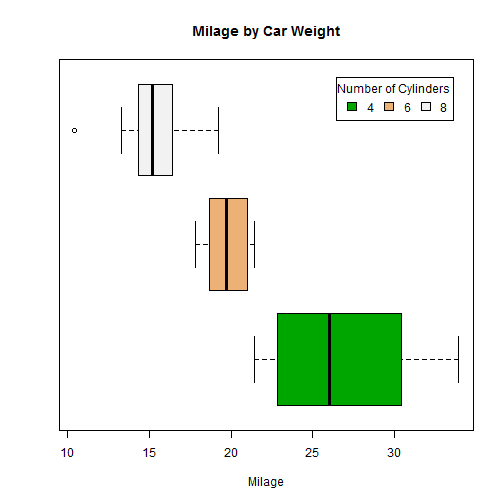# Bar plot, pie chart, histgrams and density plot, Scatterplots

| category RStudy  | tag R  Plot

### Bar plot

col = super.sym$col[1:3]), text = list(c("4 Cylinder", "6 Cylinder", "8 Cylinder"))))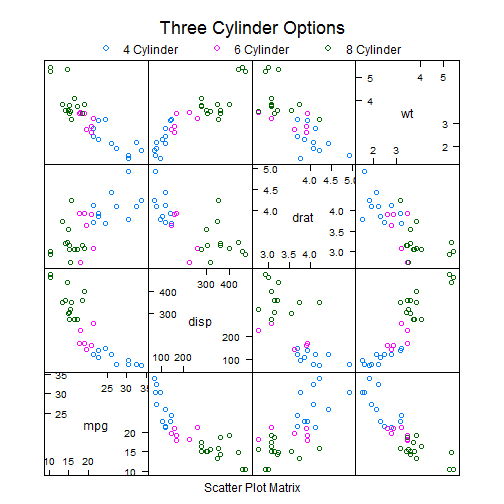#### Scatterplot Matrices from the car Package The car package can condition the scatterplot matrix on a factor, and optionally include lowess and linear best fit lines, and boxplot, densities, or histograms in the principal diagonal, as well as rug plots in the margins of the cells. library(car) scatterplot.matrix(~mpg + disp + drat + wt | cyl, data = mtcars, main = "Three Cylinder Options")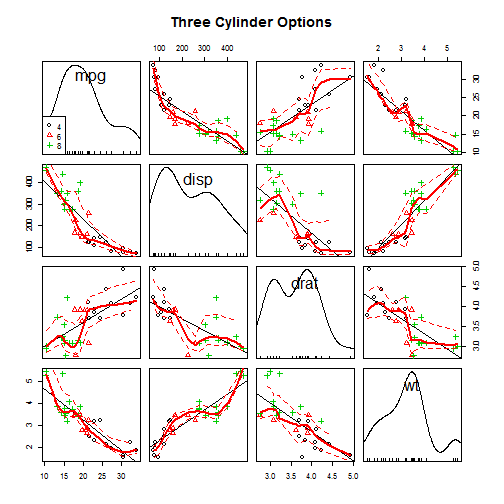#### Scatterplot Matrices from the glus Package The gclus package provides options to rearrange the variables so that those with higher correlations are closer to the principal diagonal. It can also color code the cells to reflect the size of the correlations. library(gclus) dta <- mtcars[c(1, 3, 5, 6)] # get data dta.r <- abs(cor(dta)) # get correlations dta.col <- dmat.color(dta.r) # get colors # reorder variables so those with highest correlation are closest to the # diagonal dta.o <- order.single(dta.r) cpairs(dta, dta.o, panel.colors = dta.col, gap = 0.5, main = "Variables Ordered and Colored by Correlation")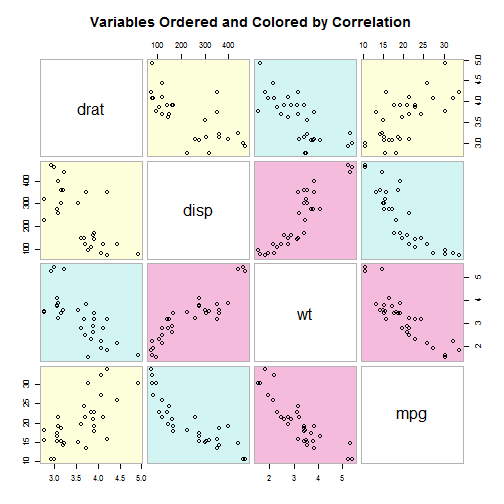### Histograms #### Simple Histogram hist(mtcars$mpg)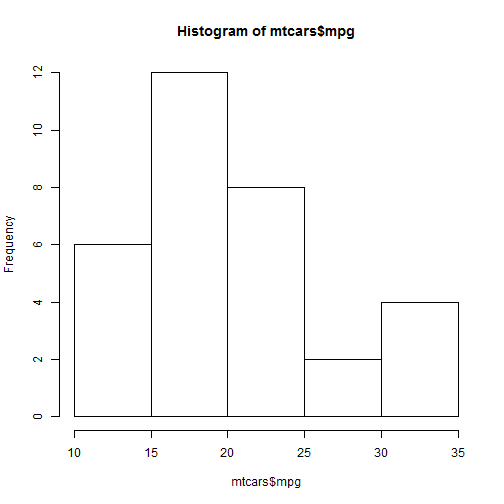hist(mtcars$mpg, breaks = 12, col = "red")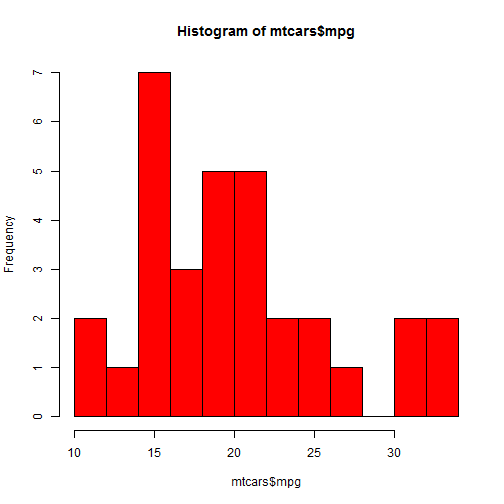#### Add a Normal Curve x <- mtcars$mpg
h <- hist(x, breaks = 10, col = "red", xlab = "Miles Per Gallon", main = "Histogram with Normal Curve")
xfit <- seq(min(x), max(x), length = 40)
yfit <- dnorm(xfit, mean = mean(x), sd = sd(x))
yfit <- yfit * diff(h$mids[1:2]) * length(x) lines(xfit, yfit, col = "blue", lwd = 2)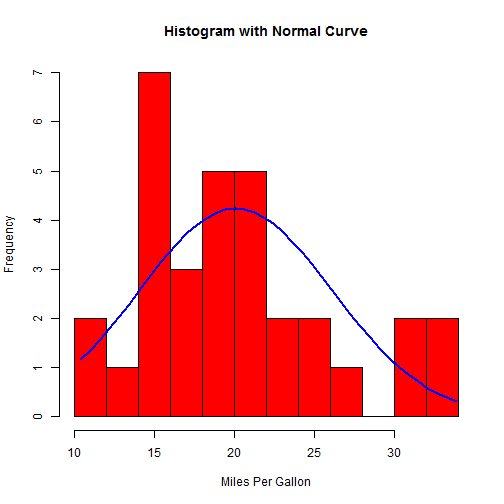### Kernel Density Plots Kernal density plots are usually a much more effective way to view the distribution of a variable. Create the plot using plot(density(x)) where x is a numeric vector. #### simple Kernel Density Plot plot(density(mtcars$mpg))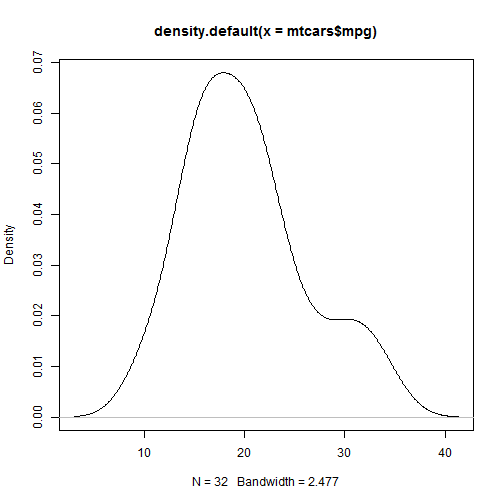d <- density(mtcars$mpg) plot(d, main = "Kernel Density of Miles Per Gallon") polygon(d, col = "red", border = "blue")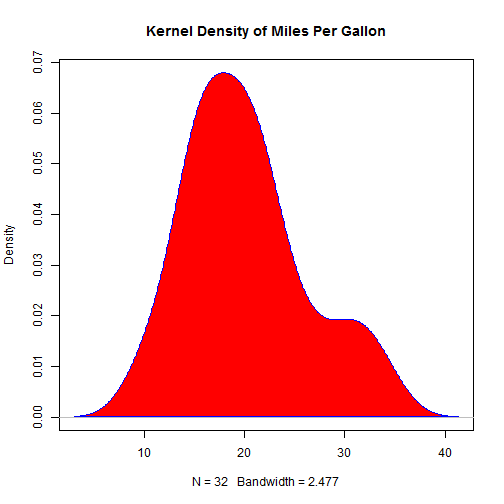### par() xaxt=”n” will suppresses plotting of the axis # par() # view current settings opar <- par() # make a copy of current settings par(col.lab = "red") # red x and y labels hist(mtcars$mpg)  # create a plot with these new settings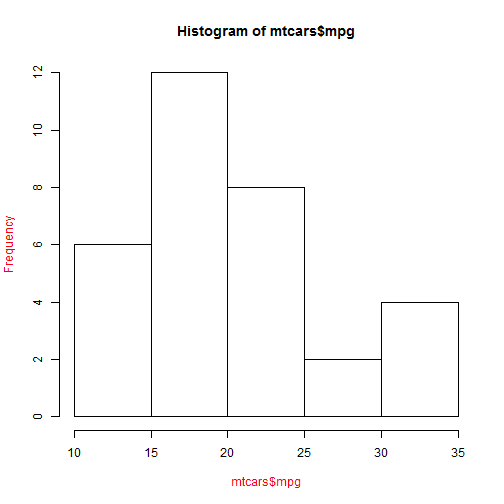par(opar)  # restore original settings


### text() and mtext()

text() places text within the graph while mtext() places text in one of the four margins.

# attach(mtcars)
plot(wt, mpg, main = "Milage vs. Car Weight", xlab = "Weight", ylab = "Mileage",
pch = 18, col = "blue")
text(wt, mpg, row.names(mtcars), cex = 0.6, pos = 4, col = "red")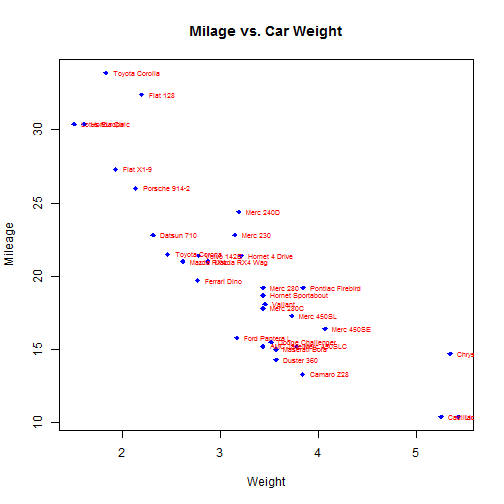### combine figures

par(mfrow = c(2, 2))
plot(wt, mpg, main = "Scatterplot of wt vs. mpg")
plot(wt, disp, main = "Scatterplot of wt vs disp")
hist(wt, main = "Histogram of wt")
boxplot(wt, main = "Boxplot of wt")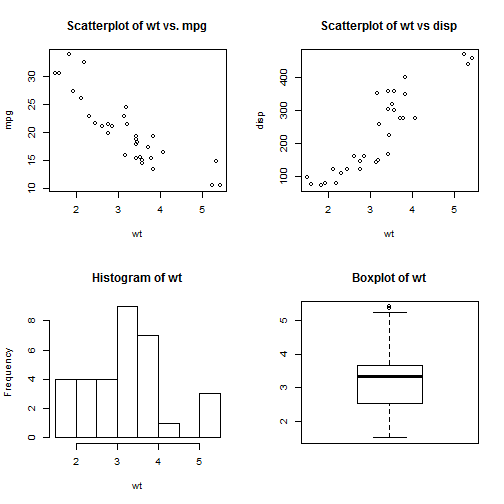# One figure in row 1 and two figures in row 2 row 1 is 1/3 the height of
# row 2 column 2 is 1/4 the width of the column 1
layout(matrix(c(1, 1, 2, 3), 2, 2, byrow = TRUE), widths = c(3, 1), heights = c(1,
2))
hist(wt)
hist(mpg)
hist(disp)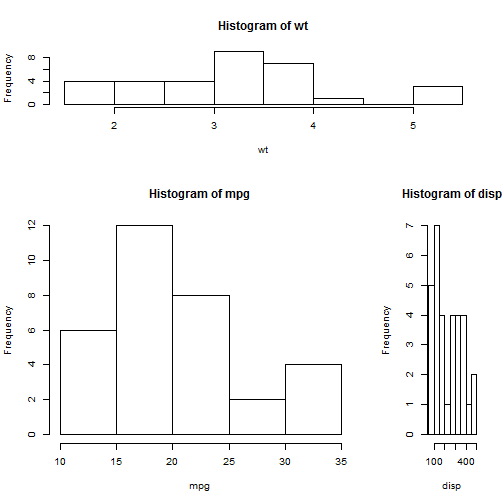# Add boxplots to a scatterplot
par(fig = c(0, 0.8, 0, 0.8), new = TRUE)
plot(mtcars$wt, mtcars$mpg, xlab = "Miles Per Gallon", ylab = "Car Weight")
par(fig = c(0, 0.8, 0.55, 1), new = TRUE)
boxplot(mtcars$wt, horizontal = TRUE, axes = FALSE) par(fig = c(0.65, 1, 0, 0.8), new = TRUE) boxplot(mtcars$mpg, axes = FALSE)
mtext("Enhanced Scatterplot", side = 3, outer = TRUE, line = -3)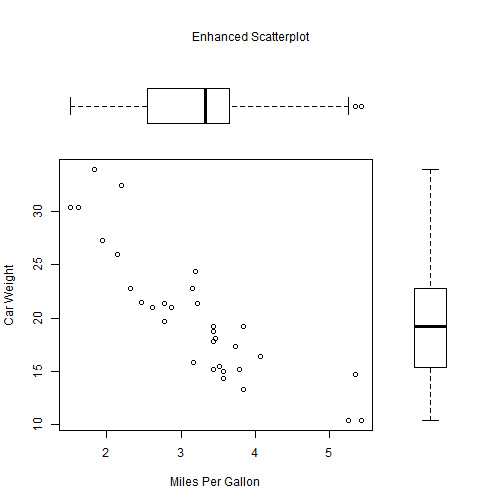To understand this graph, think of the full graph area as going from (0,0) in the lower left corner to (1,1) in the upper right corner. The format of the fig= parameter is a numerical vector of the form c(x1, x2, y1, y2). The first fig= sets up the scatterplot going from 0 to 0.8 on the x axis and 0 to 0.8 on the y axis. The top boxplot goes from 0 to 0.8 on the x axis and 0.55 to 1 on the y axis. I chose 0.55 rather than 0.8 so that the top figure will be pulled closer to the scatter plot. The right hand boxplot goes from 0.65 to 1 on the x axis and 0 to 0.8 on the y axis. Again, I chose a value to pull the right hand boxplot closer to the scatterplot. You have to experiment to get it just right.

### legend()

# attach(mtcars)
boxplot(mpg ~ cyl, main = "Milage by Car Weight", yaxt = "n", xlab = "Milage",
horizontal = TRUE, col = terrain.colors(3))
legend("topright", inset = 0.05, title = "Number of Cylinders", c("4", "6",
"8"), fill = terrain.colors(3), horiz = TRUE)